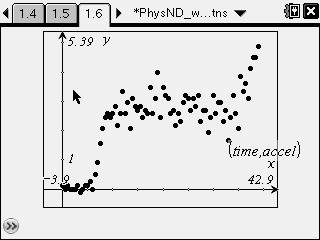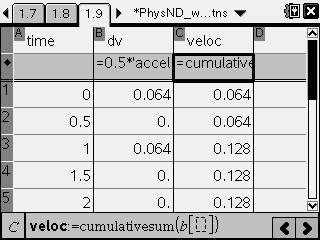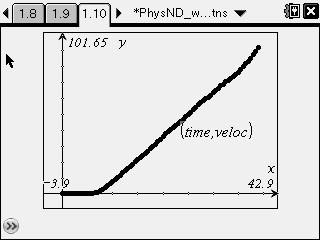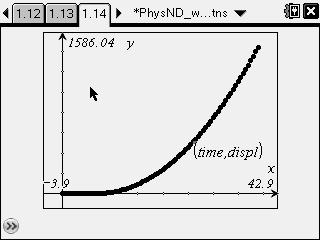# Activities

••• ##### Subject Area

• Science: Physics: Kinematics

• ##### Author9-12

45 Minutes

• ##### Device
• TI-Nspire™
• TI-Nspire™ CAS
• ##### Software

TI-Nspire™
TI-Nspire™ CAS

2.1

• ##### Report an Issue

Takeoff of an Airplane

#### Activity Overview

Students investigate the acceleration data for a taxiing Boeing 737 to calculate the velocity of the plane as it taxies down the runway.

#### Key Steps

•First, students examine a plot of the plane's acceleration and infer some of the characteristics of its motion. They make predictions about the shapes of velocity vs. time and displacement vs. time graphs for the plane.

•Next, students use the mathematical relationship between velocity and acceleration to calculate the velocity of the plane at each time point.

•Students then make a plot of calculated velocity vs. time. They compare the plot with their predictions based on acceleration.

Students then re-analyze their predictions for the graph of displacement vs. time.

•Finally, students use the mathematical relationship between displacement and velocity to calculate the displacement of the plane at each time point. They then create a graph of these data and compare it to their predictions.